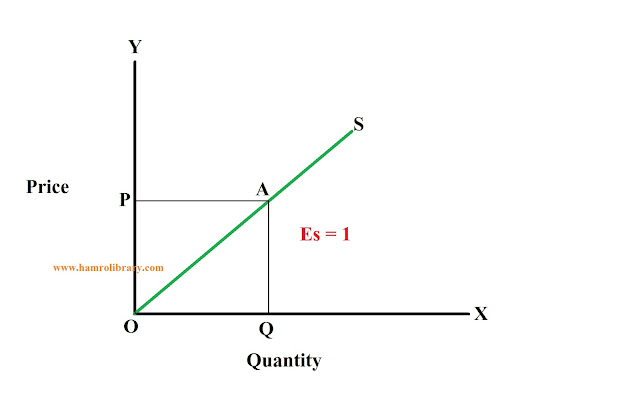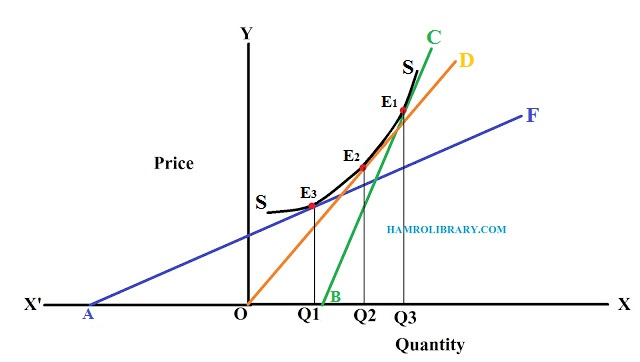Measurement of Elasticity of Supply Measurement of Elasticity of Supply

# Measurement of Elasticity of Supply

## Measurement of Elasticity of Supply

The elasticity of supply is measured on the basis of slope in the nature of the supply curve. There are three methods of measuring elasticity of supply, which are as follows:

### 1. Percentage Method:

According to this method elasticity of supply is calculated by dividing the percentage change in quantity supplied divided by the percentage change in price.

Es = Percentage change in quantity supplied / Percentage change in price

Es = ( ΔQ/ ΔP ) x (p/q)

Where,
q = initial quantity supplied
p = initial Price
ΔQ = Change in quantity supplied
ΔP = Change in price
Es = Coefficient of elasticity of supply

### 2. Arc Method

The coefficient of elasticity of supply between two points on a supply curve is called arc elasticity of supply. This method is used to measure the elasticity of supply when there is a greater change in price and quantity supplied. According to this method, the elasticity of supply is the coefficient of average between two points along a supply curve.

The figure below shows the measurement of elasticity of supply between two points A and B along the supply curve SS.

### 3. Point method

The point method is used to measure the price elasticity of supply when there is a very small change in price and quantity supplied. It is the measure of the percentage change in quantity supplied in response to a very small percentage change in price. To calculate the elasticity of supply at any point of a supply curve, the following formula is used:

Es = (ΔQ/ΔP) x (p/q)

Where,

ΔQ = Change in quantity supplied
ΔP = Change in price
p = Initial price
q = Initial quantity supplied
Es = Coefficient of elasticity of supply

The elasticity of supply depends upon the nature and slope of the supply curve, Therefore, the measurement of elasticity of supply is varied as follows:

#### i. Point elasticity on a linear supply curve:

In the figure below, supply curve SS represents the linear supply curve and P is the point where the elasticity of supply is to be measured. It shows that the initial quantity supplied is OQ at the initial price OP. When the price increases to P1Q1, the quantity supplied increases to OQ1. The supply curve SS meets at point A on the X-axis to the left from the origin.

Es at point B = (AQ/QB) X (QB/OQ)
Es at point B = AQ/OQ

The above equation shows that at point B, the price elasticity of supply is calculated by dividing AQ by OQ. Hence, at point B, Es is greater than one or relatively elastic. Hence, it can be concluded that:

a. If the supply curve meets the X-axis to the left of the origin, the price elasticity of supply will be relatively elastic.

From the figure above;
Es at point B = AQ/OQ >1 (∵ AQ> OQ)

b. If the linear supply curve passes through the origin, the price elasticity of supply will be unitary elastic or Es = 1.In the above figure, the supply curve OS is passing through the origin. The supply elasticity at point A is the ratio between OQ and OQ. Therefore, the supply elasticity at point A is unitary elastic.

Es at point A = OQ/OQ =1 (∵ OQ = OQ)

c. If the supply curve does not meet Y-axis or if it meets X-axis rightward from the origin, the price elasticity of supply will be relatively inelastic, ie. Es <1.
In the figure above, at point B, AQ is less than OQ. Therefore, the supply elasticity at point B is inelastic or less than unity. The supply elasticity at point B is the ratio between AQ & OQ, which is less than unity.

Es at point B = AQ/OQ < 1 (∵ AQ < OQ)

#### ii. Point elasticity on a non-linear supply curve

When the supply curve is a real curve, the supply elasticity is measured by drawing a line that is tangent to the supply curve. When the tangent line cuts the X-axis, the supply elasticity will be inelastic; when the tangent line cuts the Y-axis the supply elasticity will be elastic and when the tangent line passes through the origin, the supply elasticity will be unitary elastic.

The measurement of supply elasticity on a non-linear supply curve can be shown in the figure below:In the above figure, SS is a non-linear supply curve. To measure elasticity at points E1, E2,andE3, three tangents are drawn along the supply curve. i.e, BC, OD, and AF respectively. The coefficient of price elasticity at various points are as follows:

a. Es at Point E1 = BQ3/OQ3 < 1      (∵ OQ3 > BQ3)
b. Es at Point E2 = OQ2/OQ2 = 1      (∵ OQ2 = OQ2)
c. Es at Point E3 = AQ1/OQ1 > 1      (∵ AQ1 > OQ1)

### Factor influencing Elasticity of Supply

The following are the main factors, which influence the supply elasticity:

1. Nature of the commodity: The supply elasticity of durable goods is very high because durable goods can be stored for a long time, The producers are not bound to sell them in the market soon. The supply elasticity of perishable goods is low since perishable goods cannot be stored for a long time.

2. Cost of production: If production is carried out under the condition of constant return to scale, then supply can be expanded at the given price. In other words, this is a case of relatively elastic supply. If production is carried out under the condition of diminishing returns to scale (when the marginal cost rises as the production expands), then we have the case of an inelastic supply.

3. Time element: In the long run, the supply elasticity will be relatively elastic because the product can be increased in the long run. In the short run, the supply elasticity will be relatively inelastic because the productive capacity is limited.

4. Producers' expectation: If producers expect a rise in the price of a commodity in the future, they will cut down the supply in the present. As a result, the supply will be inelastic. If they expect the price to fall, in the future, they will increase the present supply. Consequently, the supply will become elastic.

5. Technical condition of production: Supply elasticity depends upon the technical condition used in the industry. In all those industries, where large plants are set up and production is carried out on a large scale, the elasticity of supply is very low because for setting up huge plants, large capital and technical expertise is required which is not easily available.

Thus, the supply of products in these industries cannot be increased easily. If the technology is simple and capital equipment is less costly, the supply of the Product will be relatively elastic.

# Second Hand Dell Laptop for Sale in Nepal

Specifications:

Brand: Dell

Display: 15.6 inches

Processor: Ryzen 7 (i7) 11th gen

RAM: 16GB DDR4

Storage: 512GB NVME SSD

Operating System: Windows 11, 64 Bit

Used for: 1 Year

Reason for Sale: Buying a Macbook.

Price: Rs. 91,000 only